# 9-gon

Sum of interiol angles of 9-gon is:

s =  1260 °

### Step-by-step explanation:

$s=180\cdot \left(9-2\right)=1260\text{°}$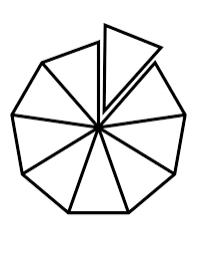Did you find an error or inaccuracy? Feel free to write us. Thank you!#### You need to know the following knowledge to solve this word math problem:

We encourage you to watch this tutorial video on this math problem:

## Related math problems and questions:

• N-gon angles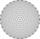What is the sum of interior angles 8-gon? What is the internal angle of a regular convex 8-polygon?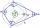What size have angles in quadrilateral (4-gon) if they are in a ratio of 8: 9: 10: 13?
• In a 2In a thirteen sided polygon, the sum of five angles is 1274°, four of the eight angles remaining are equal and the other four are 18° less than each of the equal angles. Find the angles. .
• n-gon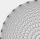Gabo draws n-gon, which angles are consecutive members of an arithmetic sequence. The smallest angle is 70° biggest 170°. How many sides have Gabo's n-gon?
• 6-gon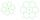Perimeter of regular hexagon is 113. Calculate its circumradius (radius of circumscribed circle).
• Six-sided polygon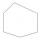In a six-sided polygon. The first two angles are equal, the third angle is twice (the equal angles), two other angles are trice the equal angle, while the last angle is a right angle. Find the value of each angle.
• Complementary angles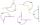Two angles are complementary. If one angle measures 10°, what is the measure of the second angle?
• Sum of inner angles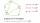Prove that the sum of all inner angles of any convex n-angle equals (n-2) . 180 degrees.
• Angles of a hexagon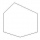Find the interior angles of a hexagon if the sizes of the angles form an arithmetic sequence, and the smallest angle is 70°.
• Area 4gonCalculate the area of 4-gon, two, and the two sides are equal and parallel with lengths 11, 5, 11, and 5. Inner angles are 45°, 135°,45°, 135°.
• Two anglesThe triangles ABC and A'B'C 'are similar. In the ABC triangle, the two angles are 25° and 65°. Explain why in the triangle A'B'C 'is the sum of two angles of 90 degrees.
• N-gon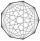How many diagonals have convex 11-gon?
• 6 regular polygonIt is given 6 side regular polygon whose side is 5 cm. Calculate its content area. Compare how many more cm2 (square centimeters) has a circle in which is inscribed the 6-gon.
• The two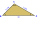The two angles of a triangle are 78° and 82°. So what is the measure of the remaining third angle?
• Regular n-gon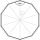In a regular n-angle polygon, the internal angle is 144 degrees. Find the number n indicating the number of sides of this polygon.
• n-gon IIWhat is the side length of the regular 5-gon circumscribed circle of radius 11 cm?
• The sum 11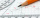The sum of the measures of two angles is 89°. One angle has a measure of 64°. What is the measure in degrees of the second angle?